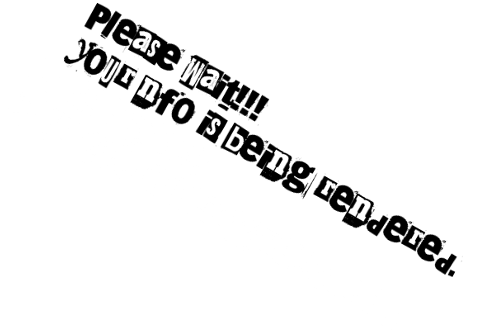NFOmation.net - Your Ultimate NFO Upload Resource! Viewing NFO file: plrus-a2bd-xqzt.nfo plrus-a2bd-xqzt```Another exquisit release

Packt.Linear.Regression.Using.Stata-XQZT

Title: Linear Regression Using Stata
Publisher: Packt
Size: 1.3G (1340408292 B)
Files: 6F
Date: 03/31/2020

Course #: 9781800207271
Type: N/A
Published: 30 Mar 2020
Modified: N/A
URL: www.packtpub.com/data/linear-regression-using-stata-video
Author: N/A
Duration: 3 hours 22 minutes
Skill: N/A
Exer/Code: [X]

Installation:
Unpack that shit, run that shit

Description:
Stata is one of the leading statistical software packages widely
used in different fields. This course is divided into two parts.
The first part covers the theory behind linear regression in an
intuitive way, and the second part enables you to apply the
theory to practical scenarios using Stata. DonΓÇÖt worry if youΓÇÖre
not from a mathematical background; the course covers only a few
equations in which addition and subtraction are
used.
YouΓÇÖll start by understanding what linear regression is and
when it is used, and then learn the differences between simple
linear regression and multiple linear regression. YouΓÇÖll get to
grips with the output of linear regression, test model
accuracy, and assumptions. YouΓÇÖll also learn how to include
different types of variables in the model, such as
youΓÇÖll use Stata to fit multiple regression models, produce
graphs that describe model fit and assumptions, and use
variable specific commands that will make the output more
readable. This part assumes basic knowledge of Stata.
By the end of
this course, youΓÇÖll have gained all the knowledge you need to
apply linear regression confidently.
All the codes and
supporting files are available at -

This NFO File was rendered by NFOmation.net```

```Another exquisit release

Packt.Linear.Regression.Using.Stata-XQZT

Title: Linear Regression Using Stata
Publisher: Packt
Size: 1.3G (1340408292 B)
Files: 6F
Date: 03/31/2020

Course #: 9781800207271
Type: N/A
Published: 30 Mar 2020
Modified: N/A
URL: www.packtpub.com/data/linear-regression-using-stata-video
Author: N/A
Duration: 3 hours 22 minutes
Skill: N/A
Exer/Code: [X]

Installation:
Unpack that shit, run that shit

Description:
Stata is one of the leading statistical software packages widely
used in different fields. This course is divided into two parts.
The first part covers the theory behind linear regression in an
intuitive way, and the second part enables you to apply the
theory to practical scenarios using Stata. Donât worry if youâre
not from a mathematical background; the course covers only a few
equations in which addition and subtraction are
used.
Youâll start by understanding what linear regression is and
when it is used, and then learn the differences between simple
linear regression and multiple linear regression. Youâll get to
grips with the output of linear regression, test model
accuracy, and assumptions. Youâll also learn how to include
different types of variables in the model, such as
youâll use Stata to fit multiple regression models, produce
graphs that describe model fit and assumptions, and use
variable specific commands that will make the output more
readable. This part assumes basic knowledge of Stata.
By the end of
this course, youâll have gained all the knowledge you need to
apply linear regression confidently.
All the codes and
supporting files are available at -

This NFO File was rendered by NFOmation.net```

aa21